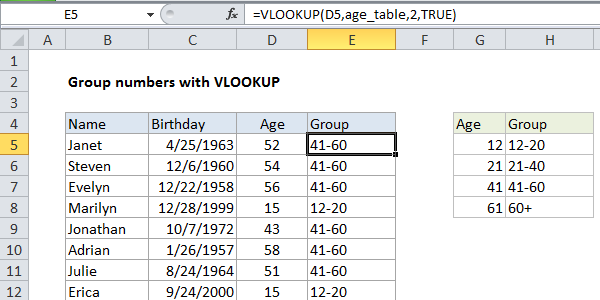## Excel Office

Excel How Tos, Tutorials, Tips & Tricks, Shortcuts

# Group numbers with VLOOKUP in Excel

This tutorial shows how to Group numbers with VLOOKUP in Excel using the example below;

## Formula

`=VLOOKUP(value,group_table,column,TRUE)`## Explanation

If you need to group by number, you can use the VLOOKUP function with a custom grouping table. This allows you to make completely custom or arbitrary groups.

Worked Example:   VLOOKUP from another workbook in Excel

In the example shown, the formula in F7 is:

`=VLOOKUP(D5,age_table,2,TRUE)`

### How this formula works

This formula uses the value in cell D5 for a lookup value, the named range “age_table” (G5:H8) for the lookup table, 2 to indicate “2nd column”, and TRUE as the last argument indicate approximate match.

Worked Example:   Lookup up cost for product or service in Excel

Note: the last argument is optional, and defaults to TRUE, but I like to explicitly set the matching mode.

VLOOKUP simply looks up the age and returns the group name from the 2nd column in the table. This column can contain any values that you wish.

Worked Example:   Extract data with helper column in Excel

### Pivot tables

Pivot tables can automatically group numbers, but the VLOOKUP approach allows you to perform completely custom grouping.